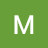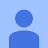Class 9 Maths ¦ ICSE ¦ Expansions ¦ Exercise 4D ¦ Selina Publications ¦ Concise Maths ¦ Maths TutorClass 9 Maths ¦ ICSE ¦ Expansions ¦ Exercise 4D ¦ Selina Publications ¦ Concise Maths ¦ Maths Tutor

9th Maths ¦ ICSE ¦ Exercise 4D ¦ Expansions ¦ Ques. No. 8,9,10 ¦ Selina Publications ¦ Maths Tutor ¦ Detailed explanation of these questions
Other questions of exercise 4D are(1-7)

algebra tutors,9th Maths,Class 9 maths,9th Math ICSE,Class 9 maths icse,9th Maths expansions,9th Maths icse ch-4 expansions,9th math ex-4D Expansions,Class 9 math Expansions exercise 4D Selina,Selina concise mathematics,9th Math ex-4D q8,9th Math ex-4D q9,9th Math ex-4D q10,Class 9 ex-4d q8 icse math,Class 9 math ex 4d Expansions selina,icse 9th Maths,icse 9th Maths ex 4d q8,Icse 9th Maths ex 4d Expansions,icse 9th Maths ex. 4D

algebratutors.org

3 Responses to “Class 9 Maths ¦ ICSE ¦ Expansions ¦ Exercise 4D ¦ Selina Publications ¦ Concise Maths ¦ Maths Tutor”

1.Micky Uppal says:

Amazing video👍

2.Raman Kakkar says:

Well done

3.Kashish X says:

Good job!!!!👍